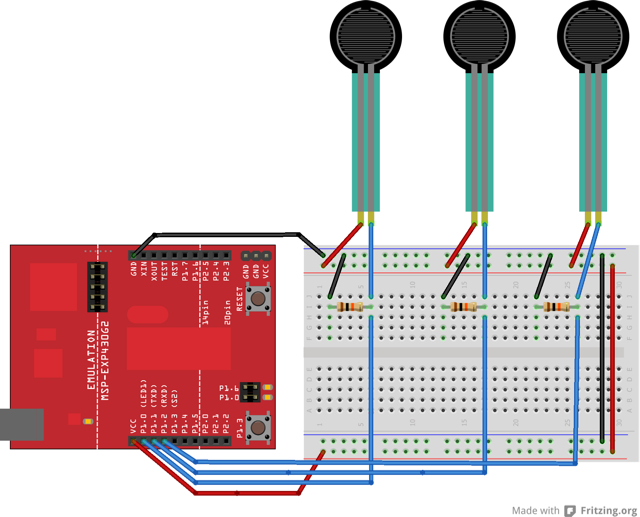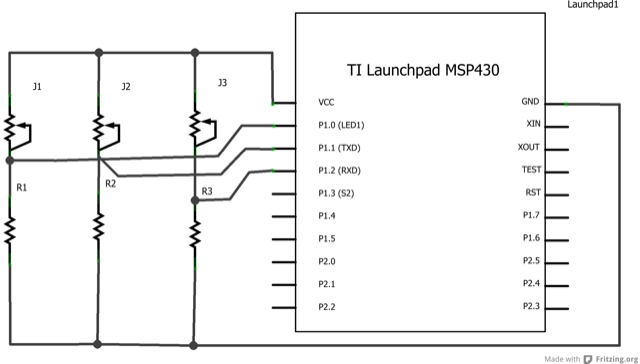## Virtual Color Mixer

This example demonstrates how to send multiple values from the MSP430 LaunchPad to the computer. The readings from three potentiometers are used to set the red, green, and blue components of the background color of a Processing sketch.

### Hardware Required

• Three potentiometers

• Three 10K ohm resistors

• Hook-up wire

### Relevant Groundwork

The sensor values are sent from the LaunchPad to the computer as ASCII-encoded decimal numbers. This means that each number is sent using the ASCII characters "0" through "9". For the value "234" for example, three bytes are sent: ASCII "2" (binary value 50), ASCII "3" (binary value 51), and ASCII "4" (binary value 52).

Connect analog sensors to analog input pins `A0, A1,` and `A2`. This circuit uses three voltage divider sub-circuits to generate analog voltages from the force-sensing resistors. A voltage divider has two resistors in series, dividing the voltage proportionally to their values.

### CircuitImage developed using Fritzing. For more circuit examples, see the Fritzing project page.

### Schematic### Code Explanation

Energia

The Energia code is straightforward for the most part. We first initialize our variables named `redPin`, `greenPin`, and `bluePin` to read the analog sensor attached to the specific pin. The `setup()` section begins the Serial communication. The `loop()` section we display the values from the analog signal on the Serial Monitor. We can check to see if the analog sensors are sending signals to the LaunchPad.

Processing

In Processing, the code generates a window that will change color according to the values set from the analog sensors. Each analog sensor is tied to a Red, Green, or Blue color and when the sensors are changed, the three colors are mixed together and the output of the sensor values will change the color on the Processing window.

### Code

```/*
This example reads three analog sensors (potentiometers are easiest)
and sends their values serially. The Processing code at the bottom
take those three values and use them to change the background color of the screen.

The circuit:
* potentiometers attached to analog inputs 0, 1, and 2

created 2 Dec 2006
by David A. Mellis
modified 30 Aug 2011
by Tom Igoe and Scott Fitzgerald
modified 16 April 2013

This example code is in the public domain.
*/

const int redPin = A0;  // sensor to control red color
const int greenPin = A1; // sensor to control green color
const int bluePin = A2; // sensor to control blue color

void setup()
{
Serial.begin(9600);
}

void loop()
{
Serial.print(",");
Serial.print(",");
}

/* Processing code for this example

// This example code is in the public domain.

import processing.serial.*;

float redValue = 0; // red value
float greenValue = 0; // green value
float blueValue = 0; // blue value

Serial myPort;

void setup() {
size(200, 200);

// List all the available serial ports
println(Serial.list());

// Open whatever port is the one you're using with the LaunchPad
myPort = new Serial(this, Serial.list(), 9600);

// don't generate a serialEvent() unless you get a newline character:
myPort.bufferUntil('\n');
}

void draw() {
// set the background color with the color values:
background(redValue, greenValue, blueValue);
}

void serialEvent(Serial myPort) {
// get the ASCII string:
String inString = myPort.readStringUntil('\n');

if (inString != null) {
// trim off any whitespace:
inString = trim(inString);
// split the string on the commas and convert the
// resulting substrings into an integer array:
float[] colors = float(split(inString, ","));
// if the array has at least three elements, you know
// you got the whole thing. Put the numbers in the
// color variables:
if (colors.length >=3) {
// map them to the range 0-255:
redValue = map(colors, 0, 1023, 0, 255);
greenValue = map(colors, 0, 1023, 0, 255);
blueValue = map(colors, 0, 1023, 0, 255);
}
}
}
*/```